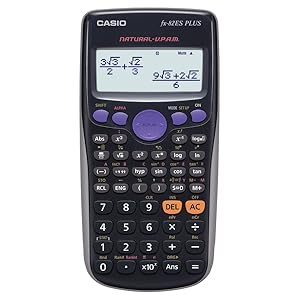-37%# Casio FX-82ES Plus Non-Programmable Scientific Calculator, 252 Functions- Open box + 1 Months RD War

625.00 399.00

Note :- Open Box

Non-Programmable Scientific Calculator with 252 Functions

Natural Textbook Display- Input and display fractions, powers, logarithms, roots, and other mathematical formulas and symbol just as they appear in textbooks

10-digit mantissa + 2-digit exponential display.

Multi-replay- Quick and easy recall of previously executed formulas for editing and re-execution.

Basic Trigonometrical & Statistics Functions

Colour coded keypad for easy key differentiation for Secondary and tertiary functions.

2 in stock

Share :

Casio fx-991ES Plus is non-programmable Scientific Calculator with 252 functions. It has Natural Textbook Display so that you can type and see algebraic functions the way it is written in your books. Calculator is powered by AAA battery. It can solve Fraction, Permutation & Combination, Statistics, Prime Factorization etc.### Features

##### New Functions
• Prime factorizations
• Random integers

Standard Functions

• Fraction calculations
• Combination and permutation
• Statistics (List-based STAT data editor, standard deviation, regression analysis)
• 9 variables
• Table function
• Comes with new slide-on hard case
• Coordinate transformation
• Power calculation
• Statistics
• Trigonometry
• Table function

# Specifications

• Non Programmable
• Number of Functions : 252
• AAA × 1 (R03)
• Natural textbook: display Display expression same as textbook
• 10 + 2 digits: 10-digit mantissa + 2-digit exponential display
• Dot matrix display: High-resolution screen provides beautiful looking graphs every time
• Multi-replay: Quick and easy recall of previously executed formulas for editing and re-execution
• Plastic keys: Designed and engineered for easy operation
• Table Generation: You can create a numerical table based on that expression by registering a function expression. In addition, graph functions can be represented by graphs
• Basic Mathematical Functions: Trigonometric, Exponential logarithmic, etc